# Difference between revisions of "SPC model of water"

The simple point charge (SPC) model  is an empirical model of water. The molecule is modelled as a rigid isosceles triangle, having charges situated on each of the three atoms. Apart from Coulombic interactions, the molecules interact via long-range Lennard-Jones sites, situated on the oxygen atoms. The parameters are as follows:

 parameter value$\sigma$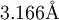$3.166 {\mathrm {\AA}}$$\epsilon$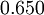$0.650$ kJ mol-1$r_\mathrm{OH}$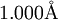$1.000\mathrm{\AA}$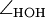$\angle_\mathrm{HOH}$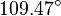$109.47^{\circ}$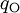$q_{\mathrm{O}}$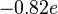$-0.82 e$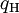$q_{\mathrm{H}}$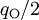$q_{\mathrm{O}}/2$ (charge neutrality)

The SPC model has a dipole moment of 2.27 D.

## Surface tension

The surface tension has been studied for the SPC model by Vega and Miguel.

## Related models

Over the years a number of variants of the SPC model have been published: